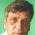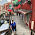## Tuesday, December 18, 2012

### Guide to Calculating the Gini Coefficient

In a previous blog I noted the amount of NCAA FBS Bowl payout inequality for the 2011 bowl season.  Here I want to provide you with a step-by-step guide using Microsoft Excel as to how I calculated the Gini coefficient using the 2011 NCAA FBS payout as an example.  This looks difficult, but taking this one step at a time, it is not.

Step 1:  Get the data you want to measure inequality.  Usually this will be income data for a population, but for illustration purposes, I used the following link for the 2011 NCAA FBS bowl payouts.  Copy and paste into Excel.

Step 2:  Sort the data.  In Excel highlight the data that you want to measure and sort from smallest to largest under Data|Sort.  (Note:  When I did this using the NCAA payout data I had to "clean" it up so that I had the payouts for both teams - just entering the same bowl and payout twice except for a few where the payouts were different for different teams - plus some general clean up to get the bowl names in column (A) and the payouts in the next column (B)).

Step 3:  Numbering the participants.  For the NCAA Bowl payout case I entered the number 1 in cell E2 and then created a formula =E2 + 1 in cell E3, and then copied this down column E for all the participants.  (Thus if there were 35 bowl games then there would be 70 teams and the last number was 70).  You could also use the fill command to do this as well.

Step 4:  Calculate n.  Next I calculated n (total number of participants).  In cell G4 I entered the following formula =countif(E2:E70,">0"), where the 70 is n in this example.

Step 5:  Calculate the cumulative percentage of the participants.  In column C, I entered the following formula:  =E2/\$G\$4, and then copy this formula down column C for all participants.  As a check, the last cell should equal 1.00.

Step 6:  Calculate the total payouts for all the participants.  In cell G6 enter the following formula:  =sum(B2:B70).

Step 7:  Calculate cumulative payout by participants.  In cell D2 I entered the following formula:  =B2/\$G\$6.  In cell D3, I entered the formula:  = D2 + B3/\$G\$6, and then copy this formula for the rest of the participants in column D.  Again as a check, the last cell should equal 1.00.

Step 8:  Calculate the participants income (or in this case payout).  In column F the calculation for the first area is different from the rest, so in cell F2 I entered the formula = 0.5*C2*D2.  In cell F3 I entered the formula = 0.5*(D2+D3)*(C3-C2).  Then copy the formula in cell F3 down column F for all the participants.

Step 9:  Calculate the total participants area.  In Excel I entered the following formula in cell G2:  =sum(F2:F100).

Step 10:  Calculate Gini Coefficient.  In cell G8, I entered the following formula:  =(0.5 - G2)/0.5 or 1-2*G2.  Both will give you the Gini Coefficient.  (If you would rather have the Gini Index - such as reported by the World Bank, just take the number calculated in cell G8 and multiply by 100.)

UPDATE (12/29/15):  corrections made in step 6 and 7 by suggestions from John Weicher.  Many thanks John.

#### 5 comments:

1.hi dear, you have just 70 observation then why have you used =sum(F2:F100) in step 9.
I have 2641 observations in my data set so kindly help me out what i have to do in step 9 i.e for F100.

2.Just change the number after the letter F to the number of rows. If the number is greater, then it has no effect as long as there is not other data below.

3.4.What if I get the negative one after step10?

1.Did you sort from lowest to highest? The Gini will not be negative.